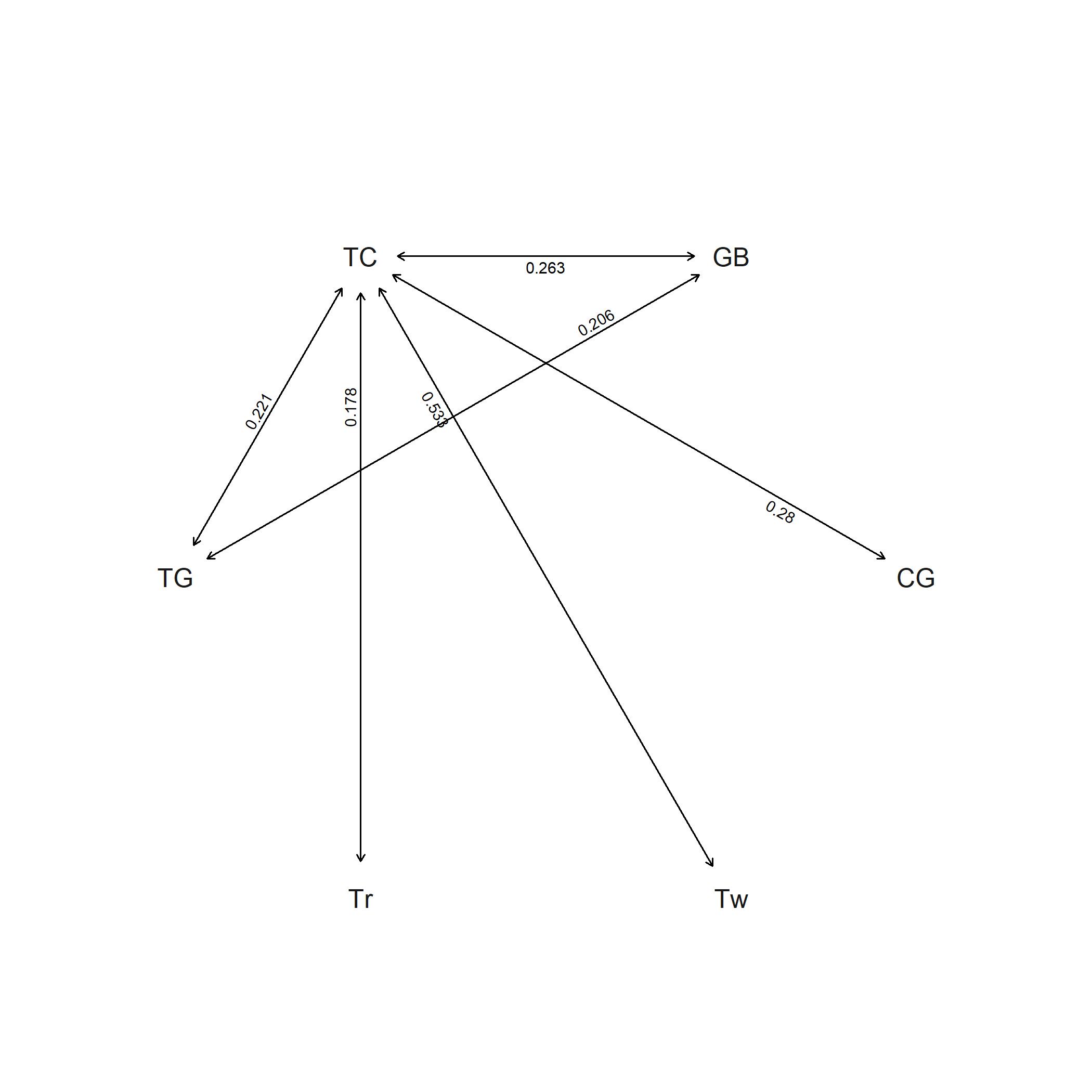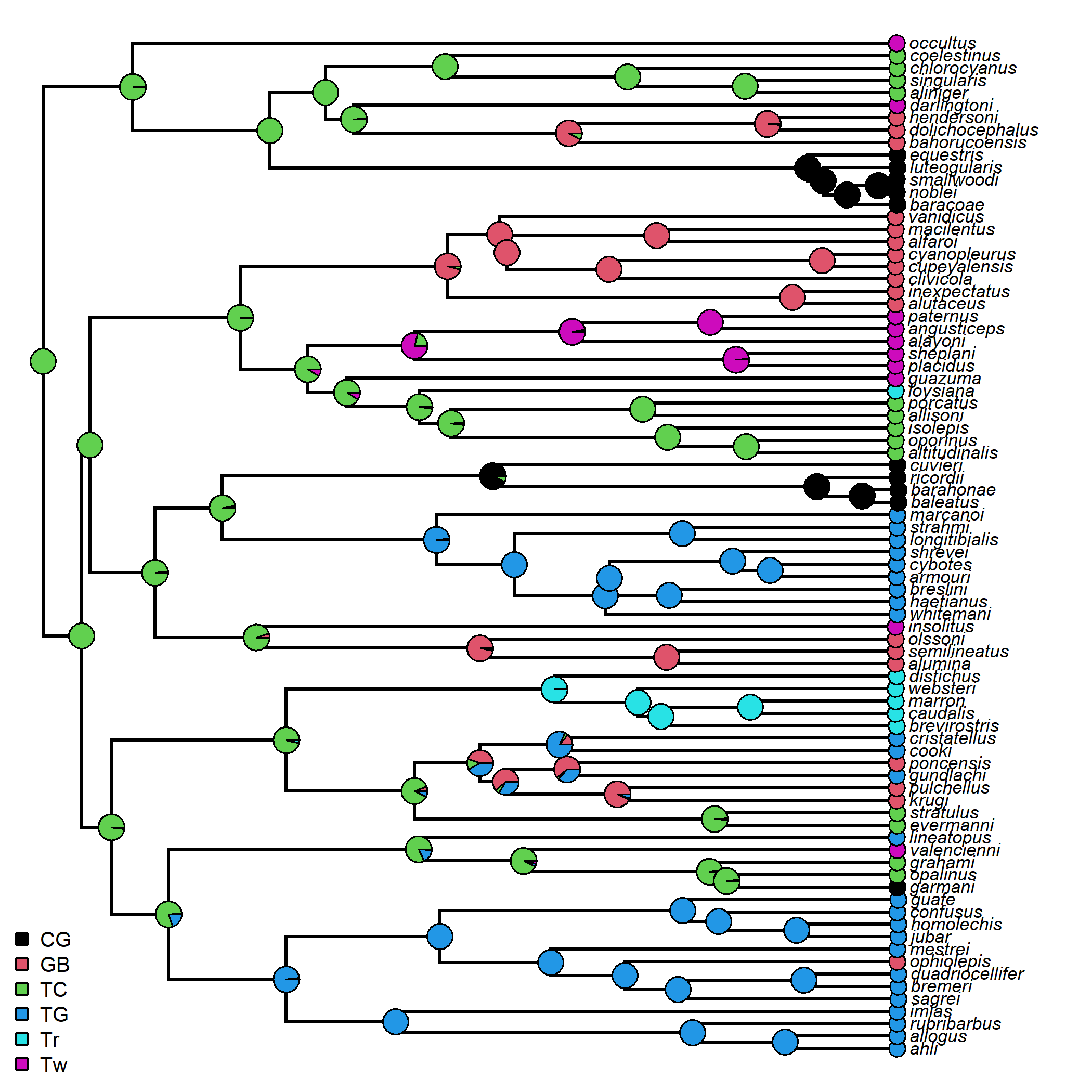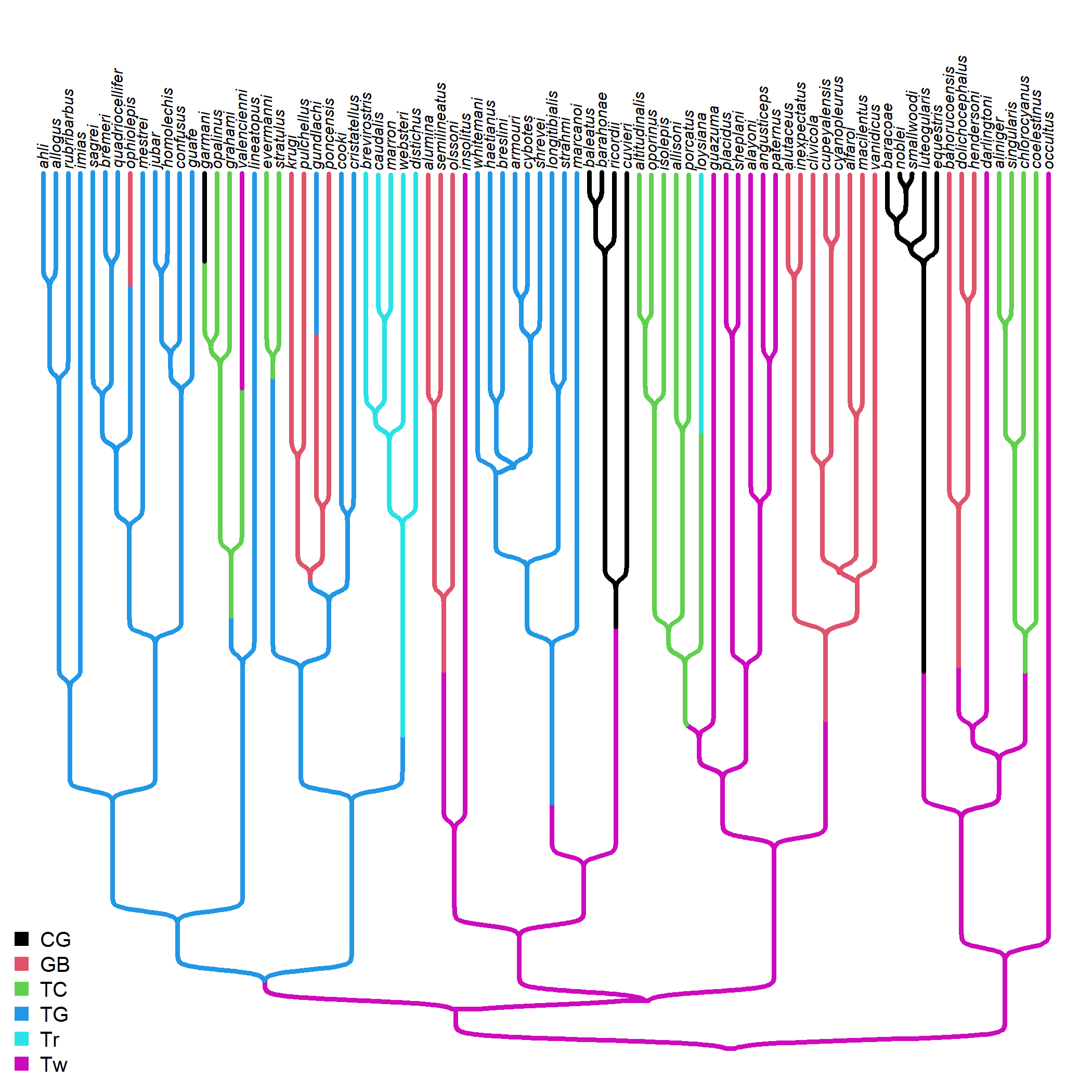## Thursday, September 1, 2022

### Stochastic character mapping in phytools with a fixed value of the Q transition matrix

Recently, a phytools user posted the following “issue” to my GitHub.

“I am working with a binary trait for which I already used a BISSE analyses, which indicated not only that the speciation rate of my taxa of interested is state-dependent but also yielded the transition rates between trait states. I was wondering if I can use that inferred transition rates from the BISSE analyses for ancestral trait reconstruction with the make.simmap function? I tried inputting it under the model but I received the following error:

``````mod<-matrix(c(0,0.005,0.216,0),2)
mtrees<-make.simmap(tree,data, model=mod, nsim=500)
Error in nlminb(q.init, function(p) lik(makeQ(m, p, index.matrix), pi = pi), :
'd' must be a nonempty numeric vector
``````

Is that a bug or am I inputting my transition rates incorrectly? Also, since I read in the manual that the values in the diagonal of the transition rate matrix will be ignored I just inserted a zero value- is that correct?”

OK, it is totally fine to pass a fitted Q matrix to `phytools::make.simmap` – but this is done using the argument `Q` not model, as indicated in the function help page:

Optional argument Q can be a string (`"empirical"` or `"mcmc"`), or a fixed value of the transition matrix, Q.

Let's try to use it in that way.

First, I'll load a phylogeny & some trait data from file.

In this case, I'll use a phylogenetic tree of Anolis lizards (of course!) and a trait vector of ecomorphological habitat specialists (“ecomorphs”).

``````library(phytools)
row.names=1)
ecomorph.tree<-drop.tip(anole.tree,
geiger::name.check(anole.tree,anole.ecomorphs)\$tree_not_data)
ecomorph<-setNames(anole.ecomorphs\$ecomorph,row.names(anole.ecomorphs))
``````

Now I'll start by fitting our model. In this case, I'll fit a simple `"SYM"` (symmetric) transition model using `geiger::fitDiscrete`. My aim is to take the estimated Q matrix from this fitted model and then pass it to `make.simmap` for stochastic mapping. (Note that I have no particular reason to imagine that `"SYM"` is a good model for this data. It's just a demo!)

``````library(geiger)
fitSYM<-fitDiscrete(ecomorph.tree,ecomorph,model="SYM")
fitSYM
``````
``````## GEIGER-fitted comparative model of discrete data
##  fitted Q matrix:
##                   CG            GB         TC            TG            Tr            Tw
##     CG -2.799773e-01  1.155981e-17  0.2799773  1.623994e-19  9.456045e-19  3.723168e-17
##     GB  1.155981e-17 -4.687714e-01  0.2628681  2.059033e-01  2.764243e-20  2.714861e-17
##     TC  2.799773e-01  2.628681e-01 -1.4756098  2.213105e-01  1.779906e-01  5.334634e-01
##     TG  1.623994e-19  2.059033e-01  0.2213105 -4.272137e-01  7.006354e-18  3.962371e-65
##     Tr  9.456045e-19  2.764243e-20  0.1779906  7.006354e-18 -1.779906e-01  1.738090e-16
##     Tw  3.723168e-17  2.714861e-17  0.5334634  3.962371e-65  1.738090e-16 -5.334634e-01
##
##  model summary:
##  log-likelihood = -71.103902
##  AIC = 172.207804
##  AICc = 179.480531
##  free parameters = 15
##
## Convergence diagnostics:
##  optimization iterations = 100
##  failed iterations = 0
##  number of iterations with same best fit = 3
##  frequency of best fit = 0.03
##
##  object summary:
##  'lik' -- likelihood function
##  'bnd' -- bounds for likelihood search
##  'res' -- optimization iteration summary
##  'opt' -- maximum likelihood parameter estimates
``````
``````plot(fitSYM,show.zeros=FALSE)
``````Next, I'm going to pull a Q matrix out of this fitted model. To do this, I'll simply using the method `as.Qmatrix`. (If this method did not exist, this step would be a lot more complicated; however, we would just need to create a k × k matrix, for k states, and then populate it with the estimated rate values from our analysis.)

``````estQ<-as.Qmatrix(fitSYM)
estQ
``````
``````## Estimated Q matrix:
##               CG            GB         TC            TG            Tr            Tw
## CG -2.799773e-01  1.155981e-17  0.2799773  1.623994e-19  9.456045e-19  3.723168e-17
## GB  1.155981e-17 -4.687714e-01  0.2628681  2.059033e-01  2.764243e-20  2.714861e-17
## TC  2.799773e-01  2.628681e-01 -1.4756098  2.213105e-01  1.779906e-01  5.334634e-01
## TG  1.623994e-19  2.059033e-01  0.2213105 -4.272137e-01  7.006354e-18  3.962371e-65
## Tr  9.456045e-19  2.764243e-20  0.1779906  7.006354e-18 -1.779906e-01  1.738090e-16
## Tw  3.723168e-17  2.714861e-17  0.5334634  3.962371e-65  1.738090e-16 -5.334634e-01
``````

Great, now let's pass this to `make.simmap`.

``````ecomorph.smap<-make.simmap(ecomorph.tree,ecomorph,Q=estQ,nsim=100)
``````
``````## make.simmap is sampling character histories conditioned on
## the transition matrix
##
## Q =
##               CG            GB         TC            TG            Tr            Tw
## CG -2.799773e-01  1.155981e-17  0.2799773  1.623994e-19  9.456045e-19  3.723168e-17
## GB  1.155981e-17 -4.687714e-01  0.2628681  2.059033e-01  2.764243e-20  2.714861e-17
## TC  2.799773e-01  2.628681e-01 -1.4756098  2.213105e-01  1.779906e-01  5.334634e-01
## TG  1.623994e-19  2.059033e-01  0.2213105 -4.272137e-01  7.006354e-18  3.962371e-65
## Tr  9.456045e-19  2.764243e-20  0.1779906  7.006354e-18 -1.779906e-01  1.738090e-16
## Tw  3.723168e-17  2.714861e-17  0.5334634  3.962371e-65  1.738090e-16 -5.334634e-01
## (specified by the user);
## and (mean) root node prior probabilities
## pi =
##        CG        GB        TC        TG        Tr        Tw
## 0.1666667 0.1666667 0.1666667 0.1666667 0.1666667 0.1666667
``````
``````## Done.
``````
``````ecomorph.smap
``````
``````## 100 phylogenetic trees with mapped discrete characters
``````

Finally, we can graph our result.

``````plot(summary(ecomorph.smap),ftype="i",fsize=0.7)
legend("bottomleft",levels(as.factor(ecomorph)),pch=22,
pt.bg=palette()[1:6],cex=0.8,pt.cex=1.2,bty="n")
``````So, that totally worked.

Now, instead, what I'm going to do is fit an equal-rates (`"ER"`) model – but then instead of using the fitted value of Q, I'm going to use a much smaller proportional matrix.

Again, this isn't something that I would necessarily recommend, but it just allows the reader to see how custom matrices can be specified.

``````fitER<-fitMk(ecomorph.tree,ecomorph,model="ER",pi="fitzjohn")
fitER
``````
``````## Object of class "fitMk".
##
## Fitted (or set) value of Q:
##           CG        GB        TC        TG        Tr        Tw
## CG -0.694496  0.138899  0.138899  0.138899  0.138899  0.138899
## GB  0.138899 -0.694496  0.138899  0.138899  0.138899  0.138899
## TC  0.138899  0.138899 -0.694496  0.138899  0.138899  0.138899
## TG  0.138899  0.138899  0.138899 -0.694496  0.138899  0.138899
## Tr  0.138899  0.138899  0.138899  0.138899 -0.694496  0.138899
## Tw  0.138899  0.138899  0.138899  0.138899  0.138899 -0.694496
##
## Fitted (or set) value of pi:
##       CG       GB       TC       TG       Tr       Tw
## 0.018212 0.203553 0.042852 0.426720 0.004400 0.304263
## due to treating the root prior as (a) nuisance.
##
## Log-likelihood: -79.19835
##
## Optimization method used was "nlminb"
``````

Now here is my Q matrix for simulation.

``````simQ<-as.Qmatrix(fitER)/100
simQ
``````
``````## Estimated Q matrix:
##              CG           GB           TC           TG           Tr           Tw
## CG -0.006944957  0.001388991  0.001388991  0.001388991  0.001388991  0.001388991
## GB  0.001388991 -0.006944957  0.001388991  0.001388991  0.001388991  0.001388991
## TC  0.001388991  0.001388991 -0.006944957  0.001388991  0.001388991  0.001388991
## TG  0.001388991  0.001388991  0.001388991 -0.006944957  0.001388991  0.001388991
## Tr  0.001388991  0.001388991  0.001388991  0.001388991 -0.006944957  0.001388991
## Tw  0.001388991  0.001388991  0.001388991  0.001388991  0.001388991 -0.006944957
``````

OK, finally, let's do our stochastic mapping!

``````map.tree<-make.simmap(ecomorph.tree,ecomorph,Q=simQ,pi="fitzjohn")
``````
``````## make.simmap is sampling character histories conditioned on
## the transition matrix
##
## Q =
##              CG           GB           TC           TG           Tr           Tw
## CG -0.006944957  0.001388991  0.001388991  0.001388991  0.001388991  0.001388991
## GB  0.001388991 -0.006944957  0.001388991  0.001388991  0.001388991  0.001388991
## TC  0.001388991  0.001388991 -0.006944957  0.001388991  0.001388991  0.001388991
## TG  0.001388991  0.001388991  0.001388991 -0.006944957  0.001388991  0.001388991
## Tr  0.001388991  0.001388991  0.001388991  0.001388991 -0.006944957  0.001388991
## Tw  0.001388991  0.001388991  0.001388991  0.001388991  0.001388991 -0.006944957
## (specified by the user);
## and (mean) root node prior probabilities
## pi =
##           CG           GB           TC           TG           Tr           Tw
## 8.245233e-06 6.364378e-03 1.380330e-05 4.211285e-03 3.066763e-07 9.894020e-01
``````
``````## Done.
``````
``````sigmoidPhylogram(map.tree,direction="upwards",lwd=3,ftype="i",
fsize=0.6)
legend("bottomleft",levels(as.factor(ecomorph)),pch=15,
col=palette()[1:6],cex=0.8,pt.cex=1.2,bty="n")
``````Woah.

Lastly, the user also asks if “the values in the diagonal of the transition rate matrix will be ignored”. OK, this is true of the design matrix (given as the argument `model`) – not of a fixed Q matrix, which should be a value Q matrix in which the diagonal is the negative row sum.

That's it!